# KSEEB SSLC Class 10 Maths Solutions Chapter 13 Statistics Ex 13.3

In this chapter, we provide KSEEB SSLC Class 10 Maths Solutions Solutions Chapter 13 Statistics Ex 13.3 for English medium students, Which will very helpful for every student in their exams. Students can download the latest KSEEB SSLC Class 10 Maths Solutions Solutions Chapter 13 Statistics Ex 13.3 pdf, free KSEEB SSLC Class 10 Maths Solutions Solutions Chapter 13 Statistics Ex 13.3 pdf download. Now you will get step by step solution to each question.

### Karnataka State Syllabus Class 10 Maths SolutionsChapter 13 Statistics Ex 13.3

Question 1.
The following frequency distribution gives the monthly consumption of electricity of 68 consumers of a locality. Find the median, mean and mode of the data and compare them.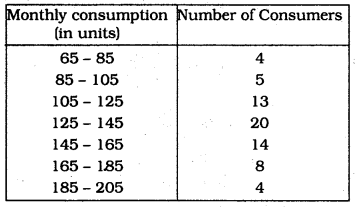Solution: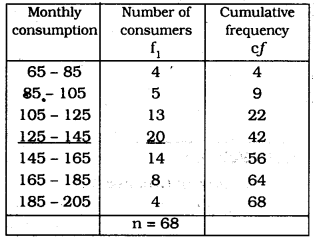(i) n = 68, ∴ n2 = 34
The median is in class interval (125 – 145)
l = 125, n = 68, f = 20, cf = 22, h = 20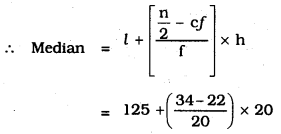= 125 + 12
∴ Median = 137 units.

(ii) To find out Mode: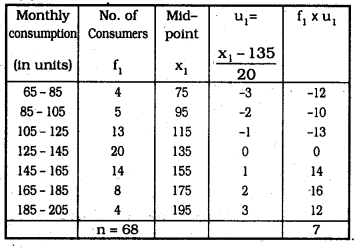Here, Mode is in class interval (125 – 145)
Maximum frequency, l = 125, f<sub>1</sub> = 20, f<sub>0</sub> = 13, f<sub>2/sub> = 14, h = 20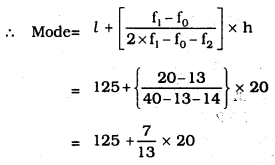= 125 + 10.76
= 135.76
∴ Mode = 135.76 units.

Question 2.
If the median of the distribution given below is 28.5, find the values of ‘x’ and ‘y’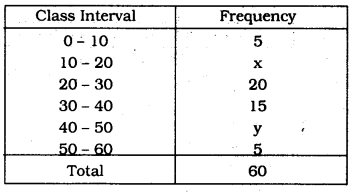Solution:
Median is 28.5
Class interval which has median is = (20 – 30)
l = 20, n = 60, f = 20, cf = 5 + a × h = 10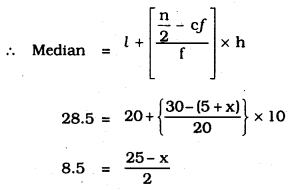17 = 25 – x
∴ x = 8
5 + x + 20 + 15 + y + 5 = 60
x + y – 45 = 60
x + y = 15
y = 15 – x
y = 15 – 8
∴ y = 7
∴ x = 8, y = 7

Question 3.
A life insurance agent found the following data for distribution of ages of 100 policy holders. Calculate the median age, if policies are given only to persons having age 18 years onwards but less than 60 year.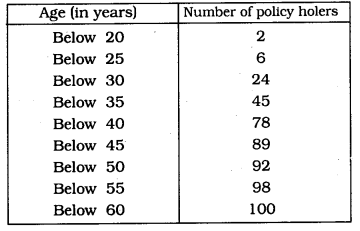Solution: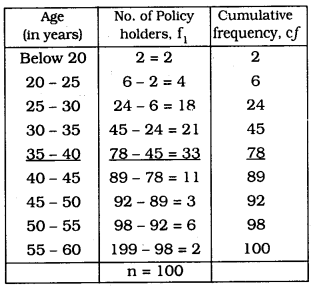n = 100 ∴ n2 = 50
Median in which C.I. is (35 – 40)
l = 35, n = 100, f = 33, cf = 45, h = 5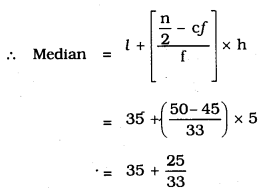= 35 + 0.76
∴ Median = 35.76 years

Question 4.
The lengths of 40 leaves of a plant are measured correct to the nearest millimetre, and the data obtained is represented in the following table: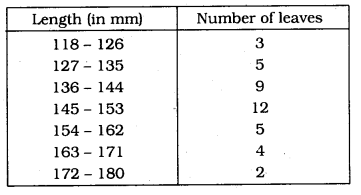Find the median length of the leaves.
(Hint: The data needs to be converted to continuous classes for finding the median, since the formula assumes continuous classes. The classes then change to 117.5 – 126.5, 126.5 – 135.5, 171.5 – 180.5)
Solution: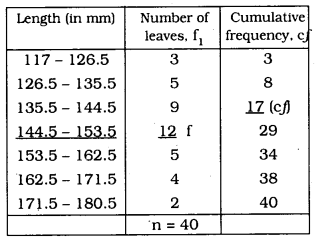n = 40 ∴ n2 = 20
C.I. which has median is (144.5 – 153.5)
l = 144.5, n = 40, f= 12, cf = 17, h = 9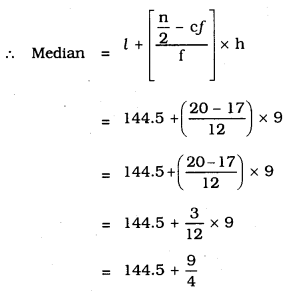= 144.5 + 2.25
∴ Median = 146.75 mm.

Question 5.
The following table gives the distribution of the lifetime of 400 neon lamps: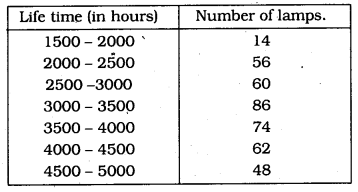Find the median life time of a lamp.
Solution: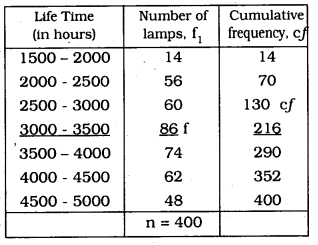n = 400 ∴ n2=4002=200
Class interval having median is = (3000 – 3500)
l = 3000, n = 400, f = 86, cf = 130, h = 500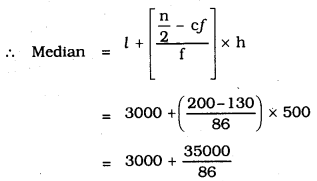= 3000 + 406.98
∴ Median = 3406.98 hours

Question 6.
100 surnames were randomly picked up from a local telephone directory and the frequency distribution of the number of letters in the English alphabets in the surnames was obtained as follows :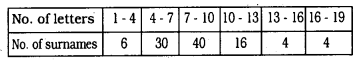Determine the median number of letters in the surnames. Find the mean number of letters in the surnames. Also, find the modal size of the surnames.
Solution: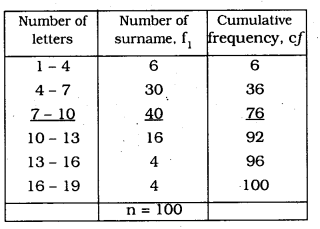(i) n = 100, ∴ n2 = 50
Class interval having median is (7 – 10)
l = 7, n = 100, f = 40, cf= 36, h = 3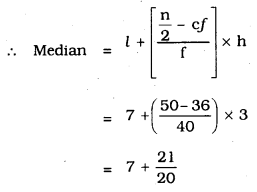= 7 + 1.05
∴ Median = 8.05 Letters

(ii) Clall interval which has mode is (7 – 10)
Maximum frequency, l = 7, f1 = 40, f0 = 30, f2 = 16, h = 3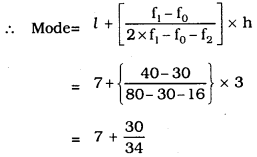= 7 + 0.88
∴ Mode = 7.88

(iii) Mean(X¯¯¯¯) : Step Deviation Method: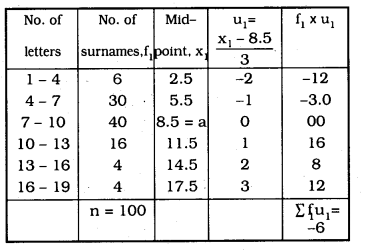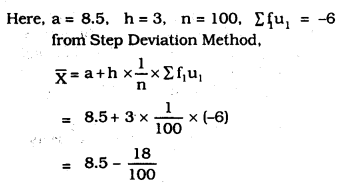= 8.5 – 0.18
= 8.32
∴ Mean = 8.32
∴ (i) Median = 8.05 letters
(ii) Mode = 7.88
(iii) Mean = 8.32.

Question 7.
The distribution below gives the weights of 30 students of a class. Find the median weight of the students.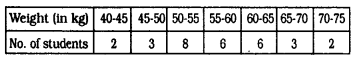Solution: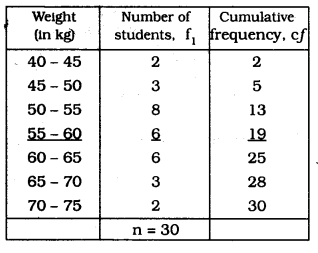(i) n = 30, ∴ n2 = 15
Class interval having median is (55 – 60)
l = 55, n = 30, f = 6, cf = 13, h = 5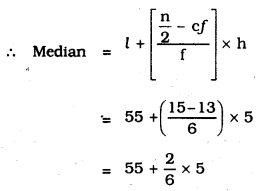= 55 + 1.67
∴ Median = 56.67 kg.

All Chapter KSEEB Solutions For Class 10 Maths

—————————————————————————–

All Subject KSEEB Solutions For Class 10

*************************************************

I think you got complete solutions for this chapter. If You have any queries regarding this chapter, please comment on the below section our subject teacher will answer you. We tried our best to give complete solutions so you got good marks in your exam.

If these solutions have helped you, you can also share kseebsolutionsfor.com to your friends.

Best of Luck!!